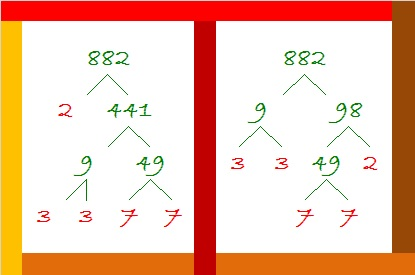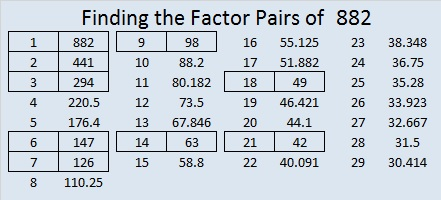# 882 Factor Trees for the First Day of Autumn

September 22 was the first day of autumn. Leaves are already beginning to fall from the trees.

To rake up the leaves for 882, you might first notice that it’s even. The logical thing to do would be to first divide 882 by two. . . But perhaps you might notice that 8 + 8 + 2 = 18, a number divisible by nine, so you might just as logically begin by dividing 882 by 9. The first step you take determines how the factor tree looks.882 has many possible factor trees but these two are probably the most common.

You can rake the leaves up this way or you can rake them up that way, but when you rake up the leaves from 882’s factor trees, you always get the same prime factors: 2, 3, 3, 7, and 7.

Here’s a little more about the number 882:

882 has eighteen factors. The greatest number less than 882 with eighteen factors is 828. Now get this: 288 also has eighteen factors. That means that every possible combination of 8-8-2 has exactly eighteen factors!

882 has some interesting representations in some other bases:

• 616 BASE 12, because 6(12²) + 1(12)¹ + 6(12º) = 882
• 242 BASE 20, because 2(20²) + 4(20)¹ + 2(20º) = 882
• 200 BASE 21, because 2(21²) = 882

882 is also the sum of consecutive primes: 439 + 443 = 882

• 882 is a composite number.
• Prime factorization: 882 = 2 × 3 × 3 × 7 × 7, which can be written 882 = 2 × 3² × 7²
• The exponents in the prime factorization are 1, 2 and 2. Adding one to each and multiplying we get (1 + 1)(2 + 1)(2 + 1) = 2 × 3 × 3 = 18. Therefore 882 has exactly 18 factors.
• Factors of 882: 1, 2, 3, 6, 7, 9, 14, 18, 21, 42, 49, 63, 98, 126, 147, 294, 441, 882
• Factor pairs: 882 = 1 × 882, 2 × 441, 3 × 294, 6 × 147, 7 × 126, 9 × 98, 14 × 63, 18 × 49 or 21 × 42
• Taking the factor pair with the largest square number factor, we get √882 = (√441)(√2) = 21√2 ≈ 29.6984848.This site uses Akismet to reduce spam. Learn how your comment data is processed.